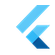Flutter Engineflutter::CounterValues Class Reference

`#include <instrumentation.h>`

## Public Member Functions

CounterValues ()

~CounterValues ()

void Visualize (SkCanvas *canvas, const SkRect &rect) const

int64_t GetCurrentValue () const

int64_t GetMaxValue () const

int64_t GetMinValue () const

## Detailed Description

Definition at line 76 of file instrumentation.h.

## ◆ CounterValues()

 flutter::CounterValues::CounterValues ( )

Definition at line 231 of file instrumentation.cc.

References ~CounterValues().

231  : current_sample_(kMaxSamples - 1) {
232  values_.resize(kMaxSamples, 0);
233 }
static const size_t kMaxSamples

## ◆ ~CounterValues()

 flutter::CounterValues::~CounterValues ( )
default

Referenced by CounterValues().

## Member Function Documentation

 void flutter::CounterValues::Add ( int64_t value )

Definition at line 237 of file instrumentation.cc.

References value.

237  {
238  current_sample_ = (current_sample_ + 1) % kMaxSamples;
239  values_[current_sample_] = value;
240 }
static const size_t kMaxSamples
uint8_t value

## ◆ GetCurrentValue()

 int64_t flutter::CounterValues::GetCurrentValue ( ) const

Definition at line 303 of file instrumentation.cc.

303  {
304  return values_[current_sample_];
305 }

## ◆ GetMaxValue()

 int64_t flutter::CounterValues::GetMaxValue ( ) const

Definition at line 307 of file instrumentation.cc.

References flutter::kMaxSamples.

Referenced by Visualize().

307  {
308  auto max = std::numeric_limits<int64_t>::min();
309  for (size_t i = 0; i < kMaxSamples; ++i) {
310  max = std::max<int64_t>(max, values_[i]);
311  }
312  return max;
313 }
static const size_t kMaxSamples

## ◆ GetMinValue()

 int64_t flutter::CounterValues::GetMinValue ( ) const

Definition at line 315 of file instrumentation.cc.

References flutter::kMaxSamples.

Referenced by Visualize().

315  {
316  auto min = std::numeric_limits<int64_t>::max();
317  for (size_t i = 0; i < kMaxSamples; ++i) {
318  min = std::min<int64_t>(min, values_[i]);
319  }
320  return min;
321 }
static const size_t kMaxSamples

## ◆ Visualize()

 void flutter::CounterValues::Visualize ( SkCanvas * canvas, const SkRect & rect ) const

Definition at line 242 of file instrumentation.cc.

References GetMaxValue(), GetMinValue(), height, flutter::kMaxSamples, flutter::path, and width.

242  {
243  size_t max_bytes = GetMaxValue();
244
245  if (max_bytes == 0) {
246  // The backend for this counter probably did not fill in any values.
247  return;
248  }
249
250  size_t min_bytes = GetMinValue();
251
252  SkPaint paint;
253
254  // Paint the background.
255  paint.setColor(0x99FFFFFF);
256  canvas->drawRect(rect, paint);
257
258  // Establish the graph position.
259  const SkScalar x = rect.x();
260  const SkScalar y = rect.y();
261  const SkScalar width = rect.width();
262  const SkScalar height = rect.height();
263  const SkScalar bottom = y + height;
264  const SkScalar right = x + width;
265
266  // Prepare a path for the data.
267  SkPath path;
268  path.moveTo(x, bottom);
269
270  for (size_t i = 0; i < kMaxSamples; ++i) {
271  int64_t current_bytes = values_[i];
272  double ratio = static_cast<double>(current_bytes - min_bytes) /
273  static_cast<double>(max_bytes - min_bytes);
274  path.lineTo(
275  x + ((static_cast<double>(i) / static_cast<double>(kMaxSamples)) *
276  width),
277  y + ((1.0 - ratio) * height));
278  }
279
280  path.rLineTo(100, 0);
281  path.lineTo(right, bottom);
282  path.close();
283
284  // Draw the graph.
285  paint.setColor(0xAA0000FF);
286  canvas->drawPath(path, paint);
287
288  // Paint the vertical marker for the current frame.
289  const double sample_unit_width = (1.0 / kMaxSamples);
290  const double sample_margin_unit_width = sample_unit_width / 6.0;
291  const double sample_margin_width = width * sample_margin_unit_width;
292  paint.setStyle(SkPaint::Style::kFill_Style);
293  paint.setColor(SK_ColorGRAY);
294  double sample_x =
295  x + width * (static_cast<double>(current_sample_) / kMaxSamples) -
296  sample_margin_width;
297  const auto marker_rect = SkRect::MakeLTRB(
298  sample_x, y,
299  sample_x + width * sample_unit_width + sample_margin_width * 2, bottom);
300  canvas->drawRect(marker_rect, paint);
301 }
DEF_SWITCHES_START snapshot asset path
Definition: switches.h:32
static const size_t kMaxSamples
int32_t width
int32_t height
int64_t GetMaxValue() const
int64_t GetMinValue() const

The documentation for this class was generated from the following files: